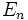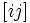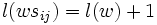# Fomin-Kirillov algebra

(diff) ← Older revision | Latest revision (diff) | Newer revision → (diff)

## Definition

The Fomin-Kirillov algebra of order$n$ over a field$k$, denoted as$E_n$, is a quadratic algebra generated (as an algebra) by elements$[ij]$ for$1 \le i \ne j \le n$ subject to the following relations:

•$[ij]^2 = 0$
•$[ij] = -[ji]$
•$[ij][km] = [km][ij]$ where all are distinct
•$[ij][jk] + [jk][ki] + [ki][ij] = 0$ for distinct$i,j,k$

## Relation with the symmetric group

The Fomin-Kirillov algebra is associated in a natural way with the symmetric group. PLACEHOLDER FOR INFORMATION TO BE FILLED IN: [SHOW MORE]

## Representations

### Bruhat representation

Further information: Bruhat representation The Bruhat representation is a representation of the Fomin-Kirillov algebra on the group algebra of the symmetric group (or rather, on the underlying module of the group algebra). In other words, we define an action of the Fomin-Kirillov algebra$E_n$ on the free module generated by the elements of the symmetric group as follows. For$i < j$:$[ij]w = ws_{ij}$ if$l(ws_{ij}) = l(w) + 1$ and 0 otherwise

Here$l$ denotes the length of the shortest word for$w \in S_n$ in terms of transpositions.

This defines an action of the Fomin-Kirillov algebra because all the defining relations of the Fomin-Kirillov algebra are satisfied by this action.

However, the representation is not faithful because there are some other relations satisfied by this action. It remains true, though, that the universal quadratic cover ofthe image is the Fomin-Kirillov algebra. This needs to be clarified/explained properly

### Divided difference operator representation

Further information: Divided difference operator representation

This is a representation mapping the Fomin-Kirillov algebra to the ring of differential operators on polynomials in$n$ variables, defined as follows:$[ij] \mapsto \partial_{ij}$

where$\partial_{ij}(f) = \frac{g - f}{x_i - x_j}$

where$g$ is obtained by interchanging the role of$x_i$ and$x_j$ in$f$.

As for the Bruhat representation, the divided difference operator representation is a representation of the Fomin-Kirillov algebra because the divided difference operators satisfy all the Fomin-Kirillov relations.

Also, like the Bruhat representation, this representation is not faithful but the Fomin-Kirillov relations are the only quadratic relations.

## APS structure

The Fomin-Kirillov algebras for different values of$n$ are closely related to each other, as follows.

There is a natural embedding$E_m \times E_n \to E_{m+n}$ which turns the sequence of Fomin-Kirillov algebras into an APS of algebras.